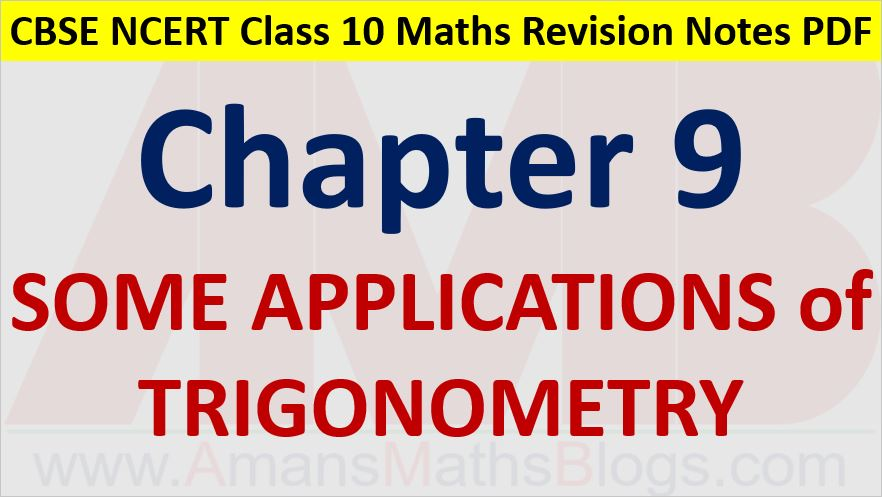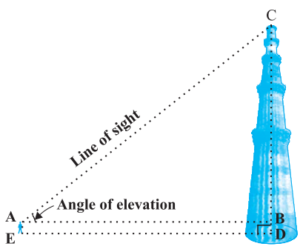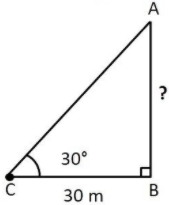Friday, October 22, 2021
Home > CBSE Class 10 > Some Applications of Trigonometry CBSE NCERT Notes Class 10 Maths Chapter 9

# Some Applications of Trigonometry CBSE NCERT Notes Class 10 Maths Chapter 9Hi students, Welcome to AMBiPi (Amans Maths Blogs). In this article, you will get Some Applications of Trigonometry CBSE NCERT Notes Class 10 Maths Chapter 9 PDF. You can download this PDF and save it in your mobile device or laptop etc.

# Some Applications of Trigonometry CBSE NCERT Notes Class 10 Maths Chapter 9 PDF

## Applications of Trigonometry

Trigonometry is used in astronomy for calculating the distances from the Earth to the planets and stars, also used in geography and in navigation,  constructing maps, determine the position of an island in relation to the longitudes and latitudes.

Read More : Real Life Application of Trigonometry

## Height and Distance

### Line of Sight

The line of sight is the line drawn from the eye of an observer to the point in the object viewed by the observer.

The angle of elevation of the point viewed is the angle formed by the line of sight with the horizontal when the point being viewed is above the horizontal level, the case when we raise our head to look at the object.

In this figure, the line AC drawn from the eye of the student to the top of the minar is called the line of sight. The angle BAC, so formed by the line of sight with the horizontal, is called the angle of elevation of the top of the minar from the eye of the student.An angle of depression of a point on the object being viewed is the angle formed by the line of sight with the horizontal when the point is below the horizontal level, i.e., the case when we lower our head to look at the point being viewed.Now, to solve the questions of applications of trigonometry (height and distance), you must need to learn concepts of trigonometry, like trigonometric ratios and trigonometric values of specific angles.

### Class 10 Maths Chapter 9 Examples 1:

The angle of elevation of the top of a tower from a point on the ground, which is 30 m away from the foot of the tower, is 30°. Find the height of the tower.tan30 = AB/BC ⇒ 1/√3 = AB/30 ⇒ AB = 30/√3 = 10√3

Click below to get CBSE Class 10 Maths Chapter wise Revision Notes PDF.

Chapter NoChapter name
Chapter 1 :Real Numbers CBSE Class 10 Maths Revision Notes
Chapter 2 :Polynomials CBSE Class 10 Maths Revision Notes
Chapter 3 :Pair of Linear Equations in Two Variables CBSE Class 10 Maths Revision Notes
Chapter 4 :Quadratic Equations CBSE Class 10 Maths Revision Notes
Chapter 5 :Arithmetic Progression CBSE Class 10 Maths Revision Notes
Chapter 6 :Triangles CBSE Class 10 Maths Revision Notes
Chapter 7 :Coordinate Geometry CBSE Class 10 Maths Revision Notes
Chapter 8 :Introduction to Trigonometry CBSE Class 10 Maths Revision Notes
Chapter 9 :Some Applications of Trigonometry CBSE Class 10 Maths Revision Notes
Chapter 10 :Circles CBSE Class 10 Maths Revision Notes
Chapter 11 :Constructions CBSE Class 10 Maths Revision Notes
Chapter 12 :Area Related to Circles CBSE Class 10 Maths Revision Notes
Chapter 13 :Surface Areas & Volumes CBSE Class 10 Maths Revision Notes
Chapter 14 :Statistics CBSE Class 10 Maths Revision Notes
Chapter 15 :Probability CBSE Class 10 Maths Revision Notes
AMBiPi
error: Content is protected !!